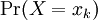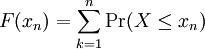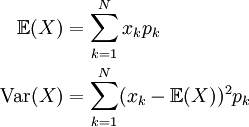# 1.9 – Lesson 1 Summary

Previous: 1.8 – Grade Distribution Example

Next: Continuous Random Variables

The treatment of discrete random variables has been brief but essential. Although the emphasis in MATH 105 is on continuous random variables, which we will study next, there are many important concepts that were introduced in Lesson 1.

## The PDF and the CDF

The probability density function (PDF) of a random variable X is the function that assigns probabilities to the possible outcomes of X. We writeto denote this function of the possible values xk of X.

The cumulative distribution function (CDF) of a random variable X is the function that accumulates the probabilities from a specified value. We define the CDF to be F(x) = Pr(Xx) and note that the CDF is intimately related to the PDF via our identity for the probability of disjoint events; i.e., the CDF is given by a sum over values of the PDF.These terms and definitions all readily extend to the case of continuous random variables. We will gain more insight and intuition into both the PDF and the CDF of a random variable as we investigate the continuous case.

## Expected Value and Variance

The concepts of an expectation and variance are crucial and will be revisited again when we explore continuous random variables. Students should know thatThe expectation represents the "centre" of a random variable, an expected value of an experiment, or the average outcome of an experiment repeated many times. The variance of a random variable is a numerical measure of the spread, or dispersion, of the PDF of X.

Previous: 1.8 – Grade Distribution Example

Next: Continuous Random Variables